# Basic Course on Turbulence and Turbulent Flow Modeling 15: 15.1 Sample calculation of a flow around a cylinder by DES

#### 15.1: Sample calculation of a flow around a cylinder by DES

We will cover a sample calculation by one of the hybrid models, DES, in this column. The objective of DES is to reduce calculation cost while capturing eddies drifting away from walls, i.e., detached eddies, in a quasi LES manner. A famous phenomenon of a vortex generated on wall and drifting away is Karman vortex in a flow around a cylinder. Karman vortex is a side-to-side vortex shedding phenomenon from a cylinder surface as shown in Figure 15.1. Since Karman vortex is a transient phenomenon in which a vortex does not stay at one place, RANS model, which is based on the calculation of averaged fields, cannot simulate it well.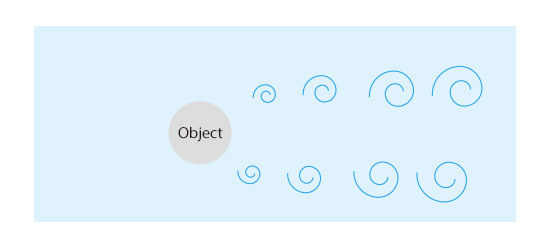Figure 15.1: Karman vortices of a flow around a cylinder

Figure 15.2 shows vortex tubes behind the cylinder calculated with the same conditions and mesh used in the sample calculation with LES in Volume 13: Methods of turbulent flow calculation (7). From top down, the results of DES, LES, and RANS model are shown. Very fine vortex tubes are generated behind the cylinder in both DES and LES. Behind them, vortex tube structures are also observed to meander widely. In the result of RANS model, on the contrary, no fine vortex tube can be found behind the cylinder. Only a series of relatively large vortices parallel to the cylinder is reproduced.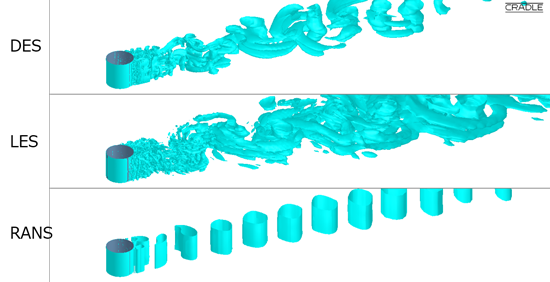Figure 15.2: Vortex tubes in a flow around a cylinder

Although DES seems to capture vortex tube distributions similar to those in LES, we find rather large differences between them with a closer look. Figure 15.3 shows enlarged views behind the cylinder in DES and LES. The looks of vortex tubes are quite different from each other between the DES on the top and LES on the bottom. In DES, most of the vortex tubes are parallel to the cylinder axis, and they form a sheet-like shape extending from the cylinder surface. In LES, on the other hand, the vortex tubes assume a more complex character than those in DES. This is probably because unsteady vortex motions near wall are not reproduced in DES, in which near-wall regions are calculated with RANS model.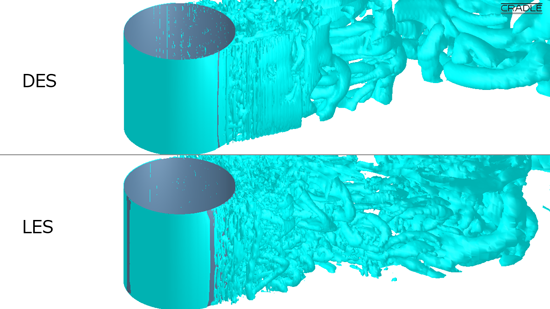Figure 15.3: Enlarged view of vortex tubes behind the cylinder

Let us look another difference between DES and LES. There is a difference in how the boundary layer on the cylinder surface develops. A boundary layer is a region formed over object’s surface, where velocity is slower than the surroundings. Figure 15.4 shows the enlarged views of the magnitude of velocity and velocity vectors near the cylinder surface. We can see that the boundary layer develops earlier in LES than in DES, and the flow separates more upstream.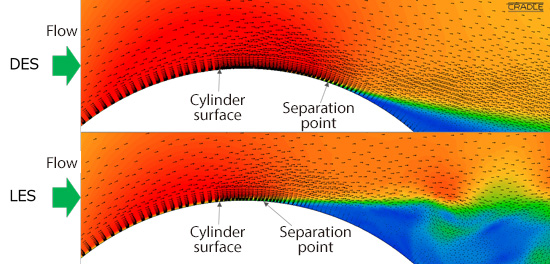Figure 15.4: Velocity profiles on the cylinder surface

Now, why are the velocity profiles near the cylinder surface different? The answer to this question can be found when we compare eddy viscosity distributions. Figure 15.5 shows the eddy viscosity distributions near the cylinder surface. In DES, regions of high eddy viscosity can be found only at the back of the cylinder (shown in green and red in the figure), whereas in LES, regions of high eddy viscosity can be found in front of the cylinder as well. That is to say, the boundary layer develops early because a large eddy viscosity is predicted. Vortex structures in DES are similar to those in LES as shown in Figure 15.2, but DES can predict only simpler vortex structures. The development of the boundary layer on the cylinder surface gives rise to this difference. Up to this column, we have looked through turbulent flow and turbulence modeling. In the next column, we will look at a sample calculation of a familiar phenomenon.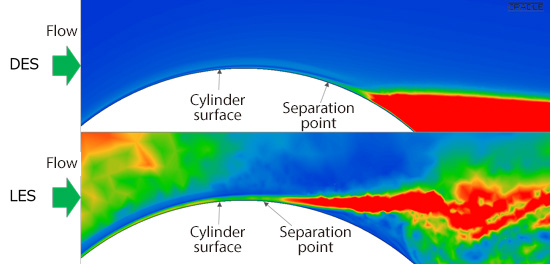Figure 15.5: Eddy viscosity near the cylinder surface